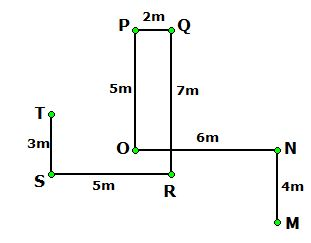# CWC/FCI Prelims 2019 Reasoning Ability Questions (Day-15)

Dear Readers, Exam Race for the Year 2019 has already started, To enrich your preparation here we are providing new series of Practice Questions on Reasoning Ability– Section for CWC/FCI Exam. Aspirants, practice these questions on a regular basis to improve your score in aptitude section. Start your effective preparation from the right beginning to get success in upcoming CWC/FCI Exam.

[WpProQuiz 5565]

Direction (1-5): Read the following information carefully and answer the questions given below.

Eight persons P, Q, R, S, T, U, V and W are born in same date and month of eight different years viz., 1972, 1975, 1979, 1984, 1988, 1994, 1997 and 2002 but not necessary in the same order. Their ages were calculated based on the year 2019.

Only three persons are born between S and Q, whose age is one of the prime number. U is not elder than Q. Only one person born between V and W, who is younger than V.As many persons born after R is same as born before V. As many persons born between W and U is same as born between T and Q. Q is not the eldest person. The age of R is not in multiples of 8.

1) Who among the following is the youngest person?

a) S

b) V

c) P

d) R

e) W

2) How many persons are born between R and W?

a) One

b) Two

c) Three

d) None

e) More than three

3) What is the difference between the ages of U and V?

a) 17 years

b) 19 years

c) 22 years

d) 6 years

e) 16 years

4) Four of the following five are alike in a certain way and hence form a group. Which one of the following that does not belong to the group?

a) U

b) P

c) W

d) R

e) S

5) Which of the following statements is true?

a) Only one person is elder than V

b) More than two persons born between Q and V

c) U was born in 1997

d) T was born immediately before Q

e) None is true

Direction (6-8): Read the following information carefully and answer the questions given below.

Eight persons R, B, V, M, K, Q, N and S are in the family of three generations. M is the only son of K. B is the son-in-law of Q, who is the mother of V. R is the paternal grandfather of N, who is the brother of S. S is the daughter of V. K is the spouse of Q.

6) How is S related to K?

a) Grandfather

b) Niece

c) Daughter

d) Granddaughter

e) None of these

7) If M is married to X, then how is X related to N?

a) Uncle

b) Sister-in-law

c) Brother-in-law

d) Aunt

e) Cannot be determined

8) How many female members are there in the family?

a) Four

b) Two

c) Three

d) Five

e) Six

Direction (9-10): Read the following information carefully and answer the questions given below.

Point S is 3m to the south of point T. Point R is 5m to the east of point S. Point Q is 7m to the north of point R. Point P is 2m to the west of point Q and 5m to the north of point O. Point N is 6m to the east of point O. Point M is 4m to the south of point N.

9) Four of the following five are alike in a certain way and hence form a group. Which one of the following that does not belong to the group?

a) TO

b) MR

c) QN

d) PM

e) TR

10) What is the direction point M with respect to point P?

a) Southwest

b) East

c) Southeast

d) Northwest

e) Northeast

Direction (1-5):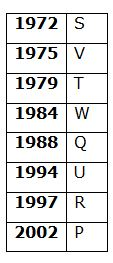• Only three persons are born between S and Q, whose age is one of the prime number. Q is not the eldest person.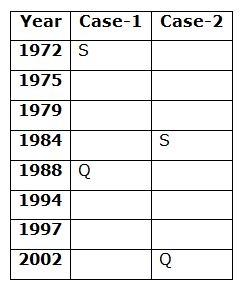• Only one person born between V and W, who is younger than V. As many persons born after R is same as born before V. The age of R is not in multiples of 8.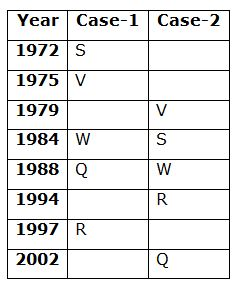• As many persons born between W and U is same as born between T and Q. U is not elder than Q.
• So, Case-2 will be dropped.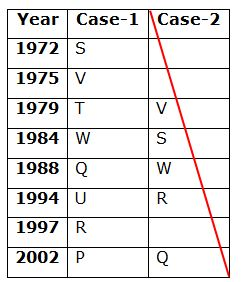Direction (6-8):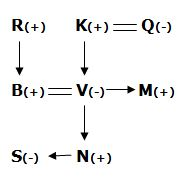Direction (9-10):